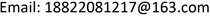Analysis of Magnetostrictive Characteristics and Vibration and Noise of Amorphous Transformer<sup> </sup>

Xiaoyu Zhou, Rui Sha, Yongheng Shi

Tiangong University, TianjinReceived: May 12th, 2021; accepted: Jun. 16th, 2021; published: Jun. 24th, 2021ABSTRACT

In order to study the magnetostrictive characteristics of amorphous alloy transformer, in this paper, the magnetic characteristics and magnetostrictive characteristics of amorphous alloy strip were measured firstly, and a prediction model for the magnetostrictive properties of amorphous alloy was established. Based on the measured data of magnetic and magnetostrictive characteristics of amorphous alloy, a finite element model of amorphous alloy transformer is established, and multi-physical field analysis is carried out. By building the magnetostrictive measurement experiments of amorphous alloy transformers at different frequencies, the magnetic characteristics and magnetostrictive characteristics at different frequencies were analyzed, and according to the measurement results, the numerical model of amorphous alloy was established for vibration and noise analysis. The accuracy of the magnetostriction prediction model is proved by comparing the measured results with the model calculation results.

Keywords:Transformer, Amorphous Alloy, Magnetostrictive Model, Vibration and Noise1. 引言

2. 非晶合金的磁致伸缩测量与模拟

2.1. 磁特性与磁致伸缩特性测量

2.2. 模型的建立

μ 0 ∫ M d H e = μ 0 ∫ M a n d H e − μ 0 ∫ k [ 1 − v ( M i r r M S ) 2 ] δ d M i r r d H e d H e − ∫ k c ( d B d t ) 2 d t − ∫ k e | d B d t | 3 / 2 d t (1)

λ = 3 2 λ S ( M M S ) 2 (2)

d λ d B = 3 λ S M M S 2 M − M a n − c δ 1 − c k [ 1 − v ( M i r r M S ) 2 ] d M a n d H e + k c d B d t + k e δ | d B d t | 1 2 μ 0 ( α − 1 ) ( M a n − M + c δ 1 − c k [ 1 − v ( M i r r M S ) 2 ] d M a n d H e ) − μ 0 δ 1 − c k [ 1 − v ( M i r r M S ) 2 ] (3)

2.3. 模型参数的计算

3. 有限元仿真模型的建立

4. 实验验证

The noise value at the measuring poin

159.660.3
256.257.2
357.658.2

5. 结论